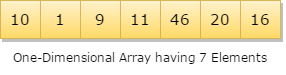## Data Structure Tutorial Index

This chapter explores various searching techniques. The process of identifying or finding a particular record is called Searching. You often spend time in searching for any desired item. If the data is kept properly in sorted order, then searching becomes very easy and efficient. In this chapter, you will get to know the basic concepts of searching that are used in the data structure and case of programming also.

## What is searching?

Searching is an operation or a technique that helps finds the place of a given element or value in the list. Any search is said to be successful or unsuccessful depending upon whether the element that is being searched is found or not. Some of the standard searching technique that is being followed in the data structure is listed below:

• Linear Search or Sequential Search
• Binary Search

## What is Linear Search?

This is the simplest method for searching. In this technique of searching, the element to be found in searching the elements to be found is searched sequentially in the list. This method can be performed on a sorted or an unsorted list (usually arrays). In case of a sorted list searching starts from 0th element and continues until the element is found from the list or the element whose value is greater than (assuming the list is sorted in ascending order), the value being searched is reached.

As against this, searching in case of unsorted list also begins from the 0th element and continues until the element or the end of the list is reached.Example:

The list given below is the list of elements in an unsorted array. The array contains ten elements. Suppose the element to be searched is '46', so 46 is compared with all the elements starting from the 0th element, and the searching process ends where 46 is found, or the list ends.

The performance of the linear search can be measured by counting the comparisons done to find out an element. The number of comparison is 0(n).

### Algorithm for Linear Search

It is a simple algorithm that searches for a specific item inside a list. It operates looping on each element O(n) unless and until a match occurs or the end of the array is reached.

• algorithm Seqnl_Search(list, item)
• Pre: list != ;
• Post: return the index of the item if found, otherwise: 1
• index <- fi
• while index < list.Cnt and list[index] != item //cnt: counter variable
• index <- index + 1
• end while
• if index < list.Cnt and list[index] = item
• return index
• end if
• return: 1
• end Seqnl_Search

## What is Binary Search?

Binary search is a very fast and efficient searching technique. It requires the list to be in sorted order. In this method, to search an element you can compare it with the present element at the center of the list. If it matches, then the search is successful otherwise the list is divided into two halves: one from the 0th element to the middle element which is the center element (first half) another from the center element to the last element (which is the 2nd half) where all values are greater than the center element.

The searching mechanism proceeds from either of the two halves depending upon whether the target element is greater or smaller than the central element. If the element is smaller than the central element, then searching is done in the first half, otherwise searching is done in the second half.

### Algorithm for Binary Search

• algorithm Binary_Search(list, item)
• Set L to 0 and R to n: 1
• if L > R, then Binary_Search terminates as unsuccessful
• else
• Set m (the position in the mid element) to the floor of (L + R) / 2
• if Am < T, set L to m + 1 and go to step 3
• if Am > T, set R to m: 1 and go to step 3
• Now, Am = T,
• the search is done; return (m)